# Effect of Coriolis force on vibration localization characteristics of mistuned bladed disk

## Hongyuan Zhang1, Huiqun Yuan2, Hongyun Sun3

1School of Automobile and Traffic, Shenyang Ligong University, Shenyang, China

1, 2, 3School of Mechanical Engineering and Automation, Northeastern University, Shenyang, China

2College of Sciences, Northeastern University, Shenyang, China

1Corresponding author

Journal of Vibroengineering, Vol. 22, Issue 5, 2020, p. 1069-1083. https://doi.org/10.21595/jve.2020.21237
Received 17 December 2019; received in revised form 5 March 2020; accepted 8 April 2020; published 15 August 2020

Copyright © 2020 Hongyuan Zhang, et al. This is an open access article distributed under the Creative Commons Attribution License, which permits unrestricted use, distribution, and reproduction in any medium, provided the original work is properly cited.
Views 118
Abstract.

Ignoring the effect of centrifugal force and Coriolis force can increase the vibration localization degree of bladed disk system. In order to address the problem of vibration localization of the mistuned bladed disk of an aeroengine, the finite element reduced order models of bladed disk was established by the component mode synthesis method. The mistuning of the blades were simulated using different mistuned modes. By analyzing the mistuned vibration localization characteristics, it was discussed whether to consider the effect of the centrifugal force and Coriolis force on the vibration localization characteristics on the bladed disk. The effect of the Coriolis force on the vibration localization of the mistuned bladed disk under different engine order of excitation was discussed. The results shows that the centrifugal force and Coriolis force strongly influences the vibration characteristics of both the tuned disk and mistuned bladed disk, in the analysis of the vibration localization characteristics of the mistuned bladed disk, the role of the Coriolis force cannot be ignored.

Highlights
• A finite element reduced order models of bladed disk based on the component mode synthesis method is proposed.
• The vibration characteristics of tuned bladed disks under different engine orders of excitation and the effect of the Coriolis force are studied.
• The vibration localization characteristics of mistuned bladed disk systems under different mistuned modes are discussed.

Keywords: mistuned bladed disk, vibration localization, centrifugal force component mode synthesis method, Coriolis force, engine order of excitation.

#### 1. Introduction

Many scholars have studied reduced-order modeling, which is based on the finite element method. Hurty and Gladwell [4, 5] established modal coordinates and proposed the concept of a modal synthesis method. Craig and Bampton  proposed the fixed interface method (C-B method), Bladh, Castanier and Pierre [7, 8] proposed the SMART (Secondary Modal Analysis Reduction) method based on the C-B method. Thus, the degree of freedom of the model was further reduced. Craig and Chang [9, 10] proposed a substructure coupling method that is based on the free interface and discussed the substructure coupling problem, which is based on improving the substructure model. He  adopted the free interface mode synthesis method for the damping vibration system to calculate the residual flexibility matrix by the weighted orthogonal matrix. The inverse of the stiffness matrix can be avoided by using the weighted orthogonal matrix. Ying  proposed a substructure mixed interface modal synthesis method with a fixed interface and a free interface. The modal synthesis technique based on these two typical modes can accurately estimate the structural modal. Based on the mixed interface method, Bai [13, 14] proposed IHISCMSM (Improved Hybrid Interface Substructural Component Modal Synthesis Method). This method can further reduce the degree of freedom after synthesis. The parametric model of the blade + disk is established by this method and based on the overall model calculation efficiency and calculation error, the results show that the computational efficiency and computational error are clearly improved compared with the classical method. Beck  proposed two methods that Component-Mode Reduced-Order Models in integrally bladed rotors, the methods provide highly accurate results in a significant reduction in solution time compared to the full finite-element model and parent reduced-order model. Based on the cyclic-based harmonic balance method, Wang and Li  established a method for solving the entire bladed disk response using a single sector matrix. This method does not have truncation errors; therefore, it provides higher solution accuracy.

Many scholars have conducted extensive research on the vibration localization of mistuned bladed disk. Ewins  considers that mistuning causes the localization phenomenon of modal vibration modes. Wei and Pierre [18, 19] studied the influence of the bladed disk parameters on mode localization. Ottarsson and Pierre  adopted the transfer matrix method and Monte Carlo simulation method to study the free vibration localization of the mistuned bladed disk. On the basis of the lumped parameter model and finite element model, given the research on the mistuned vibration characteristics of bladed disk, in order to quantitatively evaluate the modal localization caused by the mistuning, the localization factor of the modal shape is defined by Wang , Yu and Liao [21-24] to describe the localization characteristic of the mistuned bladed disk structure. Wang  studied the randomly mistuned characteristics of the group bladed disk and analysed the relationship between the mistuned sensitivity and mistuned intensity using the lumped parameter model and Monte Carlo analysis method. He and Wang [26, 27] systematically analysed the random mistuning response of the random mistuned disk system using the Monte Carlo method under different coupling strengths, especially under moderate coupling strength.

Nikolic  studied the influence of the interaction between Coriolis force and mistuned blade on the vibration characteristics of mistuned disk; furthermore, the author analysed and compared the influence of Coriolis force on the vibration of mistuned bladed disk in the case of different forced response mistuned degrees and amplitude magnification factors. Ma  adopted the ANSYS software for compressor blades to discuss the influence of centrifugal stiffening and other parameters on blade vibration characteristics.

However, the centrifugal force of the bladed disk at high speed rotation will produce a prestress effect, which is caused by the centrifugal force that has considerable influence on the natural frequency of the bladed disk. Therefore, the effect of prestress cannot be ignored in the analysis of the dynamic characteristics of the rotating machinery. In addition, the Coriolis force also has a certain influence on the natural frequency and vibration mode of the bladed disk at the high speed rotation. At present, most scholars mainly focus on the influence of the vibration characteristics when studying the Coriolis force influence on the mistuned bladed disk; therefore, fewer studies have investigated the influence of the Coriolis force on the vibration localization of the mistuned bladed disk.

On the basis of the substructures modal synthesis method for the fixed interfaces, this paper considered the effect of the centrifugal force and the Coriolis force, the component mode synthesis method for the fixed interfaces was established. Adopting this method, the finite reduced order models of the bladed disk were established. The effect of the centrifugal force and the Coriolis force on the vibration localization characteristic of the harmonic and the mistuned blades disk was discussed, and the effect of the centrifugal force and Coriolis force was analysed, which are under the different mistuned patterns and engine order of excitation on the vibration localization characteristics of the mistuned bladed disk.

#### 2. Component mode synthesis method for fixed interface

C-B method is well known for fixed interface modal synthesis method, it was proposed by Craig and Bampton. This method has been studied and improved by many scholars and has gradually matured in recent years. The substructure mode reduced-order principle of the modal synthesis method for fixed interfaces is based on two groups of modes to represent the substructure motion. One group of modes is a reduced mode set fixed for the substructure interface degree of freedom, and the other is a complete set of constraint modes that include the element deformation in an interface degree of freedom but fixed deformation in all other interface degrees of freedom. This article is based on the modal synthesis method for a fixed interface, and it’s considered the influence of the centrifugal force and Coriolis force; furthermore, this article established a finite reduced-order model of the bladed disk with the modal synthesis method for a fixed interface. The model of the bladed disk is shown in Fig. 1. The material properties of the model are listed in Table 1.

Fig. 1. The model of bladed disk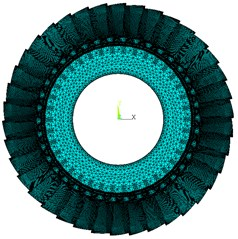a) The model of bladed disk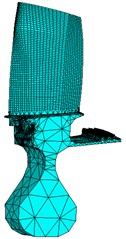b) The substructure model of bladed disk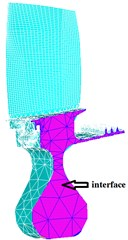c) The interface of bladed disk

Table 1. The material properties of bladed disk

 Balde material property Property value Disk material property Property value Mass density 4400 kg⋅m−3 Mass density 4700 kg⋅m-3 Modulus of elasticity 113 GPa Modulus of elasticity 150 GPa Poisson’s ratio 0.3 Poisson’s ratio 0.3

The compressor bladed disk system is assembled with 38 blades, each blade is composed of a blade body and a tenon, the blades and disk are connected through the tenon and mortise to form a bladed disk system. When the aeroengine compressor blades under centrifugal force, the natural frequency and dynamic response of the structure will be affected, considering the influence of the centrifugal stiffening effect of the rotor system on the dynamic characteristics. the equation of $i$th blade that considered the centrifugal force effect can be expressed as:

(1)
$\begin{array}{ll}{\left[M\right]}_{i}{\left\{\stackrel{¨}{X}\right\}}_{i}+{\left[C\mathrm{\text{'}}\right]}_{i}{\left\{\stackrel{˙}{X}\right\}}_{i}+{\left[K\mathrm{\text{'}}\right]}_{i}{\left\{X\right\}}_{i}={\left\{F\right\}}_{i},& \left(i=1,2,\dots ,38\right)\end{array},$

where $\left[M{\right]}_{i}$ is the mass matrix, ${\left[C\mathrm{\text{'}}\right]}_{i}$ is damping matrix, $\left[K\mathrm{\text{'}}{\right]}_{i}$ is stiffness matrix that considered centrifugal force and Coriolis force, $\left\{F{\right\}}_{i}$ is the force acting on the substructure model, and $\left\{X{\right\}}_{i}$ is the displacement vector, where:

(2)
${C}^{\mathrm{\text{'}}}=C+G,$

$C$ is damping matrix, and $G$ is Coriolis force matrix, where:

(3)
$C=\alpha M+\beta K,$
(4)
$\left\{\begin{array}{l}\alpha =\frac{4\pi {f}_{n1}{f}_{n2}\left({f}_{n1}{\xi }_{2}-{f}_{n2}{\xi }_{1}\right)}{{f}_{n1}^{2}-{f}_{n2}^{2}},\\ \beta =\frac{{f}_{n2}{\xi }_{2}-{f}_{n1}{\xi }_{1}}{\pi \left({f}_{n2}^{2}-{f}_{n1}^{2}\right)},\end{array}\right\$

where ${f}_{n1}$ and ${f}_{n2}$ are the first-order and second-order natural frequencies, respectively, ${\xi }_{1}$ and ${\xi }_{2}$ are the corresponding modal damping ratios.

The Coriolis force matrix is generated as:

(5)
$G=2\sum _{1}^{n}{\int }_{V}{N}^{T}\mathrm{\Omega }NdV,$

where $N$ is the shape function matrix; $n$ is the total number of elements; $\mathrm{\Omega }$ is the rotational matrix:

(6)
$\mathrm{\Omega }=\left[\begin{array}{ccc}0& -{\mathrm{\Omega }}_{z}& {\mathrm{\Omega }}_{y}\\ {\mathrm{\Omega }}_{z}& 0& -{\mathrm{\Omega }}_{x}\\ -{\mathrm{\Omega }}_{y}& {\mathrm{\Omega }}_{x}& 0\end{array}\right],$
(7)
${K}^{\mathrm{\text{'}}}=K+{K}_{\mathrm{c}}+{K}_{S},$

where $K$ is the stiffness matrix of the structure, ${K}_{c}$ is the centrifugal stiffening effect matrix of the rotating blades, and ${K}_{S}$ is the rotation-softening matrix.

For aeroengines and others rotating machinery that are under the effect of centrifugal force, the natural frequency and dynamic response of the rotating components will have a certain influence compared with the static equipment. Therefore, the centrifugal load should be transformed to the centrifugal force effect matrix; thus, the equation considering the centrifugal force effect is obtained.

The displacement vector $\left\{X{\right\}}_{i}$ is composed of the degree of freedom ${X}_{m}$ at the boundary and internal freedom ${X}_{s}$ of the non-interface, where $m$ is the interface DOF of the blades and disk, $s$ is the internal DOF of the blades and disk in Fig. 1, that is:

Eq. (1) can be expressed as:

(8)
${\left[\begin{array}{cc}{M}_{mm}& {M}_{ms}\\ {M}_{sm}& {M}_{ss}\end{array}\right]}_{i}{\left\{\begin{array}{c}{\stackrel{¨}{X}}_{m}\\ {\stackrel{¨}{X}}_{s}\end{array}\right\}}_{i}+{\left[\begin{array}{cc}{C\mathrm{\text{'}}}_{mm}& {C\mathrm{\text{'}}}_{ms}\\ {C\mathrm{\text{'}}}_{sm}& {C\mathrm{\text{'}}}_{ss}\end{array}\right]}_{i}\left\{\begin{array}{c}{\stackrel{˙}{X}}_{m}\\ {\stackrel{˙}{X}}_{s}\end{array}\right\}+{\left[\begin{array}{cc}{K\mathrm{\text{'}}}_{mm}& {K\mathrm{\text{'}}}_{ms}\\ {K\mathrm{\text{'}}}_{sm}& {K\mathrm{\text{'}}}_{ss}\end{array}\right]}_{i}{\left\{\begin{array}{c}{X}_{m}\\ {X}_{s}\end{array}\right\}}_{i}={\left\{\begin{array}{c}{f}_{m}\\ 0\end{array}\right\}}_{i},$

where ${f}_{m}$ is the interface force. Let the substructure of the interface be fixed, even if ${X}_{m}=0$, which can obtain:

(9)
${\left[{M}_{ss}\right]}_{i}{\left\{{\stackrel{¨}{X}}_{s}\right\}}_{i}+{\left[{C\mathrm{\text{'}}}_{ss}\right]}_{i}{\left\{{\stackrel{˙}{X}}_{s}\right\}}_{i}+{\left[{K\mathrm{\text{'}}}_{ss}\right]}_{i}{\left\{{X}_{s}\right\}}_{i}=0.$

From this Eq. (9), the normalized mode $\left[{\varphi }_{N}\right]$ is solved, that is $\left[{\varphi }_{N}\right]=\left[{\varphi }_{l},{\varphi }_{h}\right]$, where $\left[{\varphi }_{l}\right]$ –low order modal matrix, $\left[{\varphi }_{h}\right]$ – high order modal matrix.

This modal set satisfies the following conditions:

(10)
${\left[{\varphi }_{N}\right]}^{T}\left[{M}_{ss}\right]\left[{\varphi }_{N}\right]=I,$
(11)
${\left[{\varphi }_{N}\right]}^{T}\left[{K\mathrm{\text{'}}}_{ss}\right]\left[{\varphi }_{N}\right]=\left[\mathrm{\Lambda }\right]=\left[\begin{array}{ll}{\mathrm{\Lambda }}_{l}& \\ & {\mathrm{\Lambda }}_{h}\end{array}\right],$

where $\left[{\mathrm{\Lambda }}_{l}\right]=\mathrm{d}\mathrm{i}\mathrm{a}\mathrm{g}\left({\lambda }_{1}^{2},{\lambda }_{2}^{2},\cdots ,{\lambda }_{l}^{2}\right)$, $\left[{\mathrm{\Lambda }}_{h}\right]=\mathrm{d}\mathrm{i}\mathrm{a}\mathrm{g}\left({\lambda }_{l+1}^{2},{\lambda }_{l+2}^{2},\cdots ,{\lambda }_{h}^{2}\right)$, and $I$ is an identity matrix.

When analysing the vibration modal of the bladed disk, the low order mode is mainly studied, the higher order mode sets are omitted, and the lower order mode set ${\varphi }_{l}$ is selected to form the master modal set ${\varphi }_{m}$ of the substructures, that is:

(12)
$\left[{\varphi }_{m}\right]=\left[{\varphi }_{l}\right].$

If the fixed interface method [7, 8] is used, ${\varphi }_{J}$ can be expressed as follows:

(13)
$\left[{\varphi }_{J}\right]=-{{\left[{{K}^{\mathrm{\text{'}}}}_{ss}\right]}^{-1}}_{i}{\left[{{K}^{\mathrm{\text{'}}}}_{sm}\right]}_{i}\left[I\right]=-{{\left[{{K}^{\mathrm{\text{'}}}}_{ss}\right]}^{-1}}_{i}{\left[{{K}^{\mathrm{\text{'}}}}_{sm}\right]}_{i}.$

Definition $\left[{\varphi }_{c}\right]$ is the constrained modal set:

(14)
$\left[{\varphi }_{c}\right]=\left[\begin{array}{c}I\\ {\varphi }_{J}\end{array}\right]=\left[\begin{array}{c}I\\ -{{K\mathrm{\text{'}}}_{ss}}^{-1}{K\mathrm{\text{'}}}_{sm}\end{array}\right].$

The Ritz base vector of the I substructure is:

(15)
$\left[\varphi \right]=\left[{\varphi }_{m},{\varphi }_{c}\right]=\left[\begin{array}{cc}0& I\\ {\varphi }_{l}& {\varphi }_{J}\end{array}\right].$

The coordinate transformation is:

(16)
$\left[X\right]=\left[\varphi \right]\left[p\right]=\left[\begin{array}{cc}0& I\\ {\varphi }_{l}& {\varphi }_{J}\end{array}\right]\left[\begin{array}{l}{p}_{m}\\ {p}_{s}\end{array}\right].$

Eq. (16) can transform the equation of motion from physical coordinates to modal coordinates:

(17)
${\left[\stackrel{-}{M}\right]}_{i}{\left\{\stackrel{¨}{X}\right\}}_{i}+{\left[\stackrel{-}{C}\right]}_{i}{\left\{\stackrel{˙}{X}\right\}}_{i}+{\left[\stackrel{-}{K}\right]}_{i}{\left\{X\right\}}_{i}={\left\{\stackrel{-}{F}\right\}}_{i},$

where ${\left[\stackrel{-}{M}\right]}_{i}={\left[\varphi \right]}^{T}{\left[M\right]}_{i}\left[\varphi \right]$, ${\left[\stackrel{-}{C}\right]}_{i}={\left[\varphi \right]}^{T}{\left[C\mathrm{\text{'}}\right]}_{i}\left[\varphi \right]$, ${\left[\stackrel{-}{K}\right]}_{i}={\left[\varphi \right]}^{T}{\left[K\mathrm{\text{'}}\right]}_{i}\left[\varphi \right]$, ${\left[\stackrel{-}{F}\right]}_{i}={\left[\varphi \right]}^{T}{\left[F\right]}_{i}$.

The coordinates transformation of Eq. (16) uses only low order mode, ignores the high order mode, and adapts the mode truncation; therefore, the node degree of freedom is greatly reduced. The result will be constituted as the $i$ substructure formula obtained by Eq. (16):

(18)
$\begin{array}{l}\left[\begin{array}{llll}{\stackrel{-}{M}}_{1}& & & 0\\ & {\stackrel{-}{M}}_{2}& & \\ & & \ddots & \\ 0& & & {\stackrel{-}{M}}_{i}\end{array}\right]\left\{\begin{array}{l}{\stackrel{¨}{p}}_{1}\\ {\stackrel{¨}{p}}_{2}\\ ⋮\\ {\stackrel{¨}{p}}_{i}\end{array}\right\}+\left[\begin{array}{llll}{\stackrel{-}{C}}_{1}& & & 0\\ & {\stackrel{-}{C}}_{2}& & \\ & & \ddots & \\ 0& & & {\stackrel{-}{C}}_{i}\end{array}\right]\left\{\begin{array}{c}{\stackrel{˙}{p}}_{1}\\ {\stackrel{˙}{p}}_{2}\\ ⋮\\ {\stackrel{˙}{p}}_{i}\end{array}\right\}+\left[\begin{array}{llll}{\stackrel{-}{K}}_{1}& & & 0\\ & {\stackrel{-}{K}}_{2}& & \\ & & \ddots & \\ 0& & & {\stackrel{-}{K}}_{i}\end{array}\right]\left\{\begin{array}{c}{p}_{1}\\ {p}_{2}\\ ⋮\\ {p}_{i}\end{array}\right\}=\left\{\begin{array}{c}{\stackrel{-}{F}}_{1}\\ {\stackrel{-}{F}}_{2}\\ ⋮\\ {\stackrel{-}{F}}_{i}\end{array}\right\},\\ \left(i=1,2,\cdots ,38\right).\end{array}$

If the rigid connection interface is considered, through the force balance and displacement compatibility, the following can be obtained:

(19)
$\begin{array}{ll}\left\{{X}_{m1}\right\}=\left\{{X}_{m2}\right\}=\cdots =\left\{{X}_{mi}\right\},& \left(i=1,2,\cdots ,38\right)\end{array},$
(20)
$\begin{array}{ll}\left\{{f}_{m1}\right\}+\left\{{f}_{m2}\right\}+\cdots +\left\{{f}_{mi}\right\}=0,& \left(i=1,2,\cdots ,38\right)\end{array}.$

The non-independent coordinate can be transformed to the equation of motion of the generalized coordinate $\left\{q\right\}$ by the following form:

(21)

that is:

(22)
$\left\{p\right\}=\left[\beta \right]\left\{q\right\}.$

Eq. (17) is transformed to the equation of motion of the generalized coordinate by using this form $\left\{q\right\}$:

(23)
$\begin{array}{ll}{\left[\stackrel{~}{M}\right]}_{i}{\left\{\stackrel{¨}{q}\right\}}_{i}+{\left[\stackrel{~}{C}\right]}_{i}{\left\{\stackrel{˙}{q}\right\}}_{i}+{\left[\stackrel{~}{K}\right]}_{i}{\left\{q\right\}}_{i}={\left\{\stackrel{~}{F}\right\}}_{i},& \left(i=1,2,\cdots ,38\right)\end{array},$

where ${\left[\stackrel{~}{M}\right]}_{i}={\left[\beta \right]}^{T}{\left[\stackrel{-}{M}\right]}_{i}\left[\beta \right]$, ${\left[\stackrel{~}{K}\right]}_{i}={\left[\beta \right]}^{T}{\left[\stackrel{-}{K}\right]}_{i}\left[\beta \right]$, ${\left[\stackrel{~}{C}\right]}_{i}={\left[\beta \right]}^{T}{\left[\stackrel{-}{C}\right]}_{i}\left[\beta \right]$, ${\left[\stackrel{~}{F}\right]}_{i}={\left[\beta \right]}^{T}{\left[\stackrel{-}{F}\right]}_{i}$.

Eq. (23) can be adopted to solve the natural frequency of the system and the vibration mode under the generalized coordinates $\left\{q\right\}$. The vibration mode under the generalized coordinates can be returned to the physical coordinates by the coordinate transformation Eq. (23) and form Eq. (16); thus, the vibration mode of the system physical coordinates can be obtained. In practical applications, because the higher-order modals of the main interface modal $\left[\varphi \right]$ are omitted, only certain low order modals are taken; thus, the size of analyses and calculations are greatly reduced.

Based on component mode synthesis methods for fixed interface, the mistuned bladed disk is analysed. The centrifugal force produced by the centrifugal force under high speed rotation of the aeroengine compressor bladed disk system affects the natural frequency of the bladed disk. The calculate results are shown in Fig. 2 and Table 2.

Fig. 2. The natural frequencies of the bladed disk with the centrifugal force effect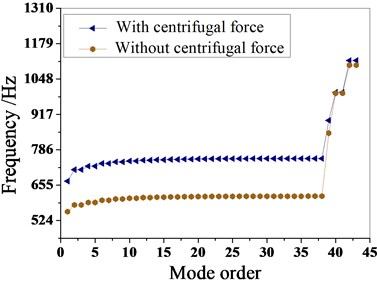Fig. 3. The effect of Coriolis force on the dynamic frequency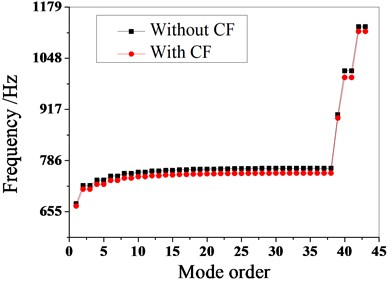Fig. 2 is the dynamic frequency comparison obtained by the modal analysis of the bladed disk Whether to consider the centrifugal force effect. It can be seen from the figure that the influence of the centrifugal force effect on the dynamic frequency of the bladed disk is remarkable. In the actual analysis, the effect of the centrifugal force on the vibration of the bladed disk cannot be ignored. From Table 2, it can be seen that whether centrifugal force is taken into account has a significant effect on the calculation accuracy, and the maximum error can reach 18.5 %, the minimum calculation error is 16.9 %. In summary, the effect of centrifugal force cannot be neglected when calculating the dynamic response of the rotating bladed disk system.

Table 2. Comparison of calculation results considering centrifugal force effect

 Mode order Dynamic frequency (Hz) Error / % Without centrifugal force With centrifugal force 1 556.88 669.8 16.9 % 2 581.98 712.62 18.3 % 3 581.98 712.62 18.3 % 4 591.07 725.38 18.5 % 5 591.07 725.38 18.5 % 6 598.96 735.35 18.5 % 7 598.96 735.35 18.5 % 8 603.85 741.16 18.5 % 9 603.85 741.16 18.5 % 10 606.9 744.79 18.5 %

The blades of the aeroengine compressor bladed disk system and other dynamic machinery are inevitably affected by the Coriolis force during operation. The influence of Coriolis force of the vibration characteristics of the bladed disk should be further studied. In this article, the influence of considering the Coriolis force on the vibration characteristics of the bladed disk is studied on the basis of the modal synthesis method for a fixed interface and finite element reduced-order models. Table 3 and Fig. 3 are the effect of the CF (Coriolis force) on the dynamic frequency.

From Fig. 3, it can be seen that the dynamic frequency of the bladed disk without considering the Coriolis force effect is larger than that of the bladed disk considering the Coriolis force softening slightly rotation effect, and it can be seen that the Coriolis force effect can reduce the dynamic frequency of the disk system. From Table 3, it can be seen that the calculation error caused by the effect of the Coriolis force is not more than 2 %. Although the error is small, for aeroengine compressor bladed disk system, a small difference in the amplitude of each blade can cause vibration localization, Therefore, the effect of the Coriolis force on the vibration characteristics of a bladed disk system cannot be neglected in the analysis of dynamic characteristics.

Table 3. Comparison of calculation results with Coriolis force on the dynamic frequency

 Mode order Dynamic frequency (Hz) Error / % Without Coriolis With Coriolis 1 675.27 669.8 0.8 % 2 721.84 712.62 1.3 % 3 721.84 712.62 1.3 % 4 736 725.38 1.5 % 5 736 725.38 1.5 % 6 746.47 735.35 1.5 % 7 746.47 735.35 1.5 % 8 752.64 741.16 1.5 % 9 752.64 741.16 1.5 % 10 756.51 744.79 1.6 %

#### 3. Effect of Coriolis force on vibration localization characteristics of mistuned bladed disk

The equation of tuned bladed disk can be expressed as:

(24)
$M\stackrel{¨}{X}+C\stackrel{˙}{X}+KX=F.$

Under the circumstance of only considering stiffness mistuning, the kinetic equation of the mistuned bladed disk can be expressed as:

(25)
$M\stackrel{¨}{X}+C\stackrel{˙}{X}+\left(K+\mathrm{\Delta }K\right)X=F,$

where $\mathrm{\Delta }K$ is the stiffness mistuning matrix, and the $i$-th component of the travelling wave exciting force $F$ can be expressed as:

(26)

where ${F}^{0}$ is the vibration amplitude of the exciting force load by $i$th rotor blade, $\omega$ is the excitation frequency, ${\phi }_{i}$ is the phase angle of the travelling wave exciting force on the $i$th blade, as follows:

(27)

where $E$ is the engine order of excitation.

#### 3.1. Effect of Coriolis force on vibration characteristics of tuned bladed disk

Under differents engine order of excitation, by calculating whether to consider the effect of the Coriolis force on the amplitude of the tuned bladed disk, the results shown in Fig. 4 are obtained. The figure shows that the maximum amplitude of the bladed disk without considering the Coriolis force effect is larger than with it. Regardless of whether to consider softening, the maximum amplitude of the tuned bladed disk tends to gradually increase with the increase of the engine order of excitation.

Fig. 4. The maximum amplitude of the tuned bladed disk with and without Coriolis force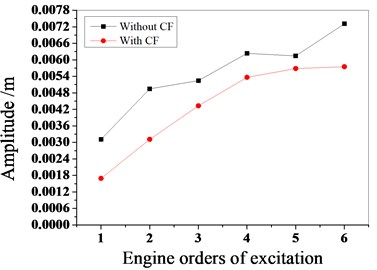Fig. 5. Vibration characteristics of tuned bladed disk with and without Coriolis Force when $E$ is 4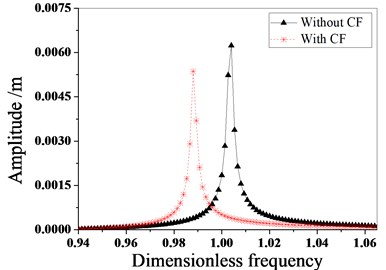a) Amplitude frequency characteristics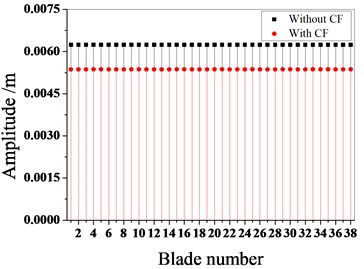Fig. 5 illustrates whether to consider the influence of the Coriolis force on the amplitude frequency characteristics of the bladed disk when the engine order of excitation is 4. As shown in the figure, when considering softening, the frequency of the maximum amplitude of the tuned bladed disk decreases, and the maximum amplitude also decreases considerably. It can be seen that the Coriolis effect influences the frequency characteristics of the tuned bladed disk. Fig. 6 is the distribution of deformation in the case of whether or not the Coriolis force is taken into account.

Fig. 6. Deformation distribution of tuned bladed disk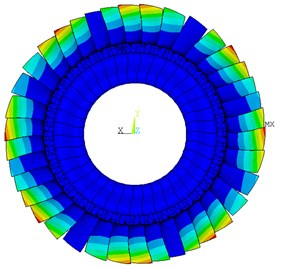a) Without Coriolis force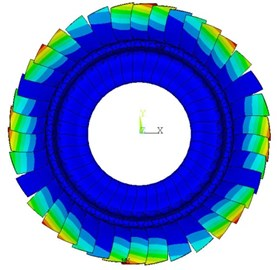b) With Coriolis force

#### 3.2. Effect of Coriolis force on vibration localization characteristics of mistuned bladed disk

In order to analyze the effect of the Coriolis force on the vibration localization of mistuned bladed disk, two typical mistuned modes and three random mistuned modes are used to simulation analysis.

Fig. 7. Typical mistuning value of blades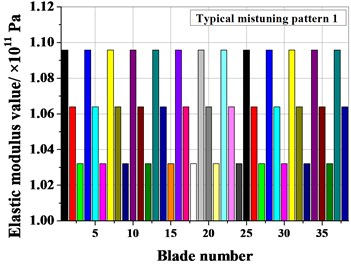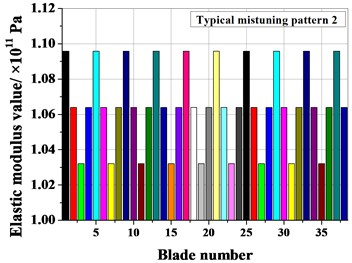Fig. 8. Amplitude frequency characteristics of mistuned bladed disk with and without Coriolis force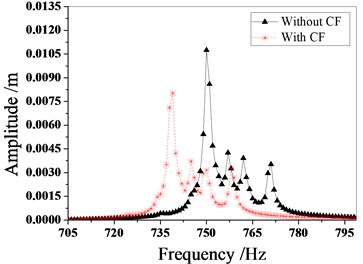a) Typical mistuning pattern 1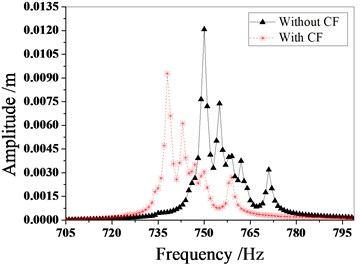b) Typical mistuning pattern 2

Fig. 7 shows the typical mistuning value of the blades, and Fig. 8 shows the vibration response characteristics of the typical mistuning bladed disk system with or without considering the Coriolis force. Fig. 9 shows the effect of the Coriolis force on the maximum amplitude of the typical mistuned blade. Figs. 10 and 11 show the effect of the Coriolis force on the amplitude distribution of two typical mistuned pattern.

Fig. 9. Maximum amplitude of blades with and without Coriolis force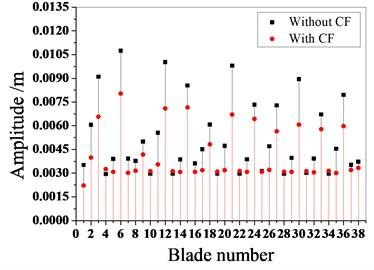a) Typical mistuning pattern 1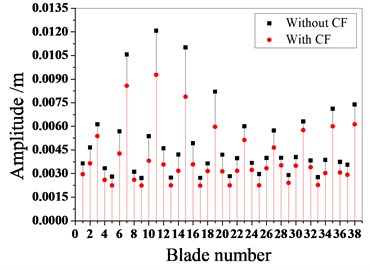b) Typical mistuning pattern 2

Fig. 10. Deformation distribution of typical mistuning pattern 1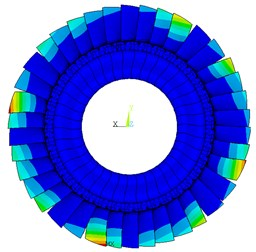a) Without Coriolis force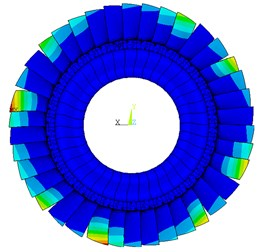b) With Coriolis force

Fig. 11. Deformation distribution of typical mistuning pattern 2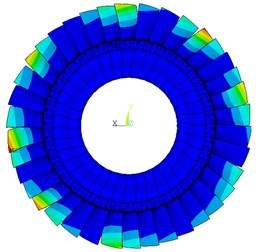a) Without Coriolis force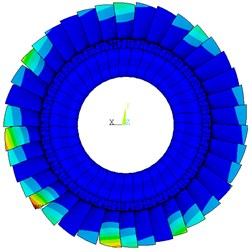b) With Coriolis force

When considering only stiffness mistuning, the blade shows mistuning according to the experimental data. The blade mistuning value of the three types of mistuned patterns is obtained, as shown in Fig. 12, by the mistuned identification for the elastic modulus of the blade.

Fig. 13 and Fig. 14 are the amplitude frequency characteristics of the bladed disk under three mistuned patterns and the maximum amplitude distribution of each blade. It can be seen from the figure that regardless of the mistuned patterns, the amplitude of mistuned bladed disk is small with Coriolis force. The resonance frequency also decreases, but the trend of the amplitude frequency characteristic curve is the same.

Fig. 12. Mistuning value of blades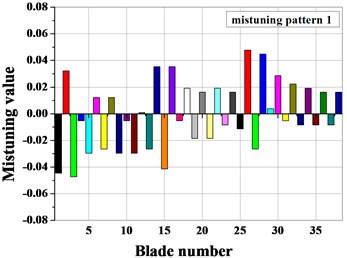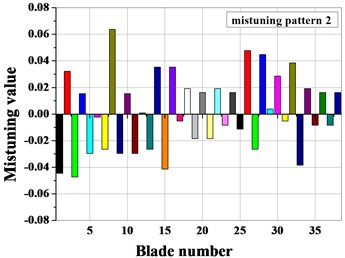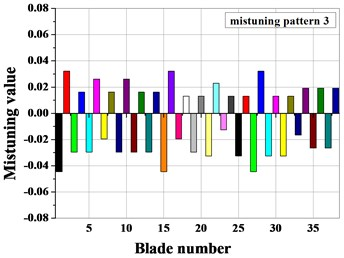Fig. 13. Amplitude frequency characteristics of mistuned bladed disk with and without Coriolis force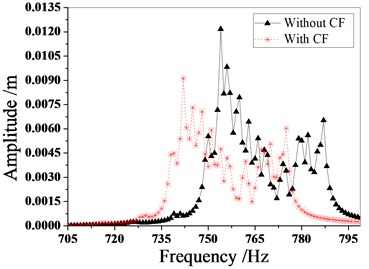a) Mistuned pattern 1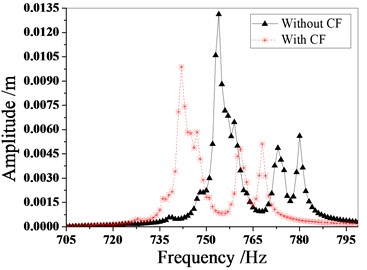b) Mistuned pattern 2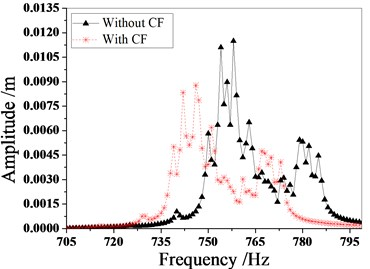c) Mistuned pattern 3

Fig. 14. Maximum amplitude of blades with and without Coriolis forcea) Mistuned pattern 1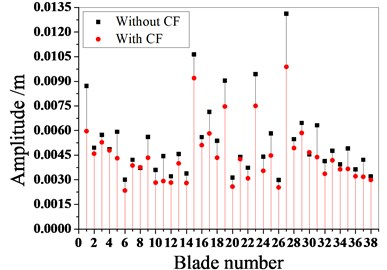b) Mistuned pattern 2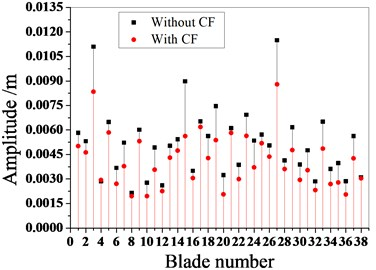c) Mistuned pattern 3

Fig. 15. The vibration localization characteristics of the mistuned bladed disk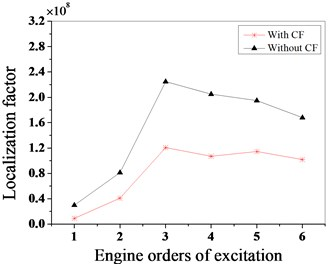a) Mistuned pattern 1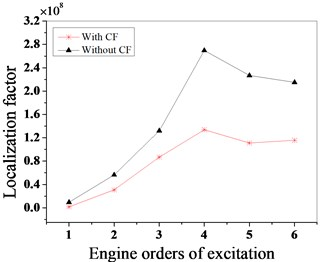b) Mistuned pattern 2c) Mistuned pattern 3

The vibration localization factor in the literature  is an important indicator to evaluate the vibration characteristics of mistuned bladed disk. To analyze the influence of the Coriolis force on the vibration localization of the mistuned bladed disk, the vibration localization factor is adopted. The below equation for calculating this factor:

(28)
$L=\mathrm{m}\mathrm{e}\mathrm{a}\mathrm{n}\left(X\right)×\mathrm{v}\mathrm{a}\mathrm{r}\left(X\right),$

where, $X$ – maximum amplitude vector of each blade, $\mathrm{m}\mathrm{e}\mathrm{a}\mathrm{n}\left(X\right)$ is the mean of maximum amplitude of each blade, $\mathrm{v}\mathrm{a}\mathrm{r}\left(X\right)$ is the maximum amplitude variance of each blade.

The vibration localization characteristics of the bladed disk under three mistuned patterns are analyzed by this factor. As shown in Fig. 15, the maximum localization factor appears in the mistuned pattern 1 when the engine order of excitation is 3, 4 in mistuned pattern 2; and 5 in mistuned pattern 3. When considering the Coriolis force effect, the localization degree of the bladed disk is lower than without considering it.

#### 4. Conclusions

This paper is the first to describe the effect of the Coriolis force effect on the vibration localization of a mistuned bladed disk. The mistuned bladed disk model was established by the mode synthesis method for fixed interface. By analyzing the effect of the centrifugal force and Coriolis force on the vibration localization characteristics of the mistuned bladed disk, the significance of this study to engineering and conclusions as follow:

1) The calculation problem with a large number of elements and nodes can be solved by the mode synthesis method for fixed interface, this method can greatly reduce the calculation time.

2) The centrifugal force has a significant effect on the calculation results, and the maximum error can reach 18.5 %. The minimum calculation error is 16.9 %. When considering the centrifugal force effect, the vibration frequency of the bladed disk is larger than without consideration, and the effect of the centrifugal force cannot be ignored when the dynamic response of the rotation bladed disk is calculated.

3) Although the calculation error of the dynamic frequency of the bladed disk caused by the Coriolis force is not more than 2 %, but it has a significant effect on the vibration localization of mistuned bladed disk system.

4) The Coriolis force has a different effect on the vibration localization degree of the bladed disk system with the engine order excitation and mistuned patterns. This paper provides a method for engineering calculation to analyze the localization degree under different mistuning modes and engine order excitation.

#### Acknowledgements

This research is supported by project of the National Natural Science Foundation of China (No. 51775093), China Postdoctoral Science Foundation (2019M651147), Doctoral Research Initiation Fund of Liaoning Provincial (2020-BS-156), Shenyang Youth Science and Technology Innovation Talent Project (RC200006) and Scientific Research Fund Project of Liaoning Provincial Department of Education (LG202008).

1. Csaba G. Forced response analysis in time and frequency domains of a tuned bladed disk with friction dampers. Journal of Sound and Vibration, Vol. 214, Issue 3, 1998, p. 395-412. [Publisher]
2. Zeng H. N., Cao S. Q., Su Y. L. Vibration localization of a mistuned bladed disk system with friction and gap. Journal of Vibration and Shock, Vol. 35, Issue 2, 2016, p. 82-90. [Search CrossRef]
3. Liu Y. L., Shangguan B., Xu Z. L. Anti-aliasing alternating frequency/time domain method and its application in response analysis of blade systems. Journal of Aerospace Power, Vol. 27, Issue 6, 2012, p. 1238-1242. [Search CrossRef]
4. Hurty W. C. Vibrations of structural systems by component mode synthesis. Journal of the Engineering Mechanics Division, Vol. 86, Issue 4, 1960, p. 51-69. [Publisher]
5. Gladwell G. M. L. Branch mode analysis of vibrating systems. Journal of Sound and Vibration, Vol. 1, Issue 1, 1964, p. 41-59. [Publisher]
6. Craig R. R., Bampton M. D. D. Coupling of substructures for dynamic analyses. AIAA Journal, Vol. 6, Issue 7, 1968, p. 1313-1319. [Publisher]
7. Bladh R., Castanier M. P., Pierre C. Component-mode-based reduced order modeling techniques for mistuned bladed disks-part I: theoretical models. Journal of Engineering for Gas Turbines and Power, Vol. 123, Issue 1, 2000, p. 89-99. [Publisher]
8. Bladh R., Castanier M. P., Pierre C. Component-mode-based reduced order modeling techniques for mistuned bladed disks – part II: Application. Journal of Engineering for Gas Turbines and Power, Vol. 123, Issue 1, 2000, p. 100-108. [Publisher]
9. Craig R. R., Chang C. J. Free-interface methods of substructure coupling for dynamic analysis. AIAA Journal, Vol. 14, Issue 11, 1976, p. 1633-1635. [Publisher]
10. Craig R. R., Chang C. J. Substructure Coupling for Dynamic Analysis and Testing. Report NASA-CR-2781, 1977, p. 389-392. [Search CrossRef]
11. He H., Chen G. P., Lu Y. H. Free-interface component mode synthesis methods for damped vibration systems. Chinese Journal of Computational Mechanics, Vol. 26, Issue 6, 2009, p. 951-955. [Search CrossRef]
12. Ying Z. G., Qiu J. B. Hybrid modal synthesis techniques based on free interfacial and fixed interfacial substructural modes and its application. Chinese Journal of Computational Mechanics, Vol. 14, Issue 1, 1997, p. 64-68. [Search CrossRef]
13. Bai B., Bai G. C., Li C. Application of improved hybrid interface substructural component modal synthesis method in vibration characteristics of mistuned blisk. Chinese Journal of Mechanical Engineering, Vol. 51, Issue 9, 2015, p. 73-81. [Publisher]
14. Bai B., Bai G. C., Li C., Zhao H. Y., Yao W. Vibratory characteristic analysis of integral mistuned bladed disk assemblies for aeroengine. Proceedings of the Institution of Mechanical Engineers Part C Journal of Mechanical Engineering Science, Vol. 229, Issue 16, 2015, p. 2921-2938. [Publisher]
15. Beck J. A., Brown J. M., Cross C. J., Slater J. C. Component-mode reduced-order models for geometric mistuning of integrally bladed rotors. AIAA Journal, Vol. 52, Issue 7, 2014, p. 1345-1356. [Publisher]
16. Wang P. Y., Li, L. Reduced order computational method for analysis of mistuning bladed disk dynamics characteristic. Journal of Aerospace Power, Vol. 29, Issue 6, 2014, p. 1395-1402. [Search CrossRef]
17. Ewins D. J., Imregun M. Vibration modes of packeted bladed disks. ASME Journal of Vibration, Acoustics, Stress and Reliability in Design, Vol. 106, 1984, p. 175-180. [Publisher]
18. Wei S. T., Pierre C. Localization phenomena in mistuned assemblies with cyclic symmetry part 2: forced vibrations. ASME Journal of Vibration, Acoustics, Stress and Reliability in Design, Vol. 110, Issue 4, 1988, p. 439-449. [Publisher]
19. Pierrre C., Murthy D. V. Aeroelastic modal characteristics of mistuned blade assemblies-mode localization and loss of eigenstructure. AIAA Journal, Vol. 30, Issue 10, 1992, p. 2483-2496. [Publisher]
20. Ottarsson G., Pierre C. A Transfer matrix approach to free vibration localization in mistuned bladed assemblies. Journal of Sound and Vibration, Vol. 197, Issue 5, 1996, p. 589-618. [Publisher]
21. Wang J. J., Yao J. Y., Li Q. H. Probability characteristics of vibratory mode of bladed disk assemblies with random stiffness mistuning. Journal of Aerospace Power, Vol. 23, Issue 2, 2008, p. 256-262. [Search CrossRef]
22. Yu C. B., Wang J. J., Li Q. H. Probability characteristics for response localization of mistuned bladed disk assemblies. Journal of Aerospace Power, Vol. 25, Issue 9, 2010, p. 2006-2012. [Search CrossRef]
23. Liao H. T. Wang J. J., Li Q. H. Mistuning characteristics analysis of mistuned bladed disk assemblies. Journal of Aerospace Power, Vol. 25, Issue 1, 2010, p. 160-168. [Search CrossRef]
24. Liao H. T., Wang J. J., Li Q. H. Mistuned forced response characteristics analysis of mistuned multi-stages bladed disks. Journal of Vibration and Shock, Vol. 30, Issue 3, 2011, p. 22-29. [Search CrossRef]
25. Wang A. L., Sun B. H. Analysis of natural vibration localization of random mistuned bladed disk with grouped blades. China Mechanical Engineering, Vol. 22, Issue 7, 2011, p. 771-775. [Search CrossRef]
26. He E. M., Yu S. X., Wang H. J. Statistical analysis of the forced response of random mistuning bladed disk. Journal of Mechanical Strength, Vol. 29, Issue 1, 2007, p. 20-24. [Search CrossRef]
27. Wang H. J., He E. M., Zhao Z. B. Investigating further resonant response characteristics of disk with randomly mistuned blades. Journal of Northwestern Polytechnical University, Vol. 29, Issue 2, 2011, p. 189-193. [Search CrossRef]
28. Nikolic M., Petrov E. P., Ewins D. J. Coriolis forces in forced response analysis of mistuned bladed disks. Journal of Turbomachinery, Vol. 129, 2007, p. 730-739. [Publisher]
29. Ma H., Sun Q., Tai X. Y., Hao Y. M., Wen B. C. Vibration response analysis on the rotating blade-casing rubbing. Journal of Vibration and Shock, Vol. 36, Issue 14, 2017, p. 26-32. [Search CrossRef]
30. Yuan H. Q., Zhang L., Han Q. K., Song L. Optimization of mistuning blades arrangement for vibration absorption in an aero-engine based on artificial ant colony algorithm. Journal of Vibration and Shock, Vol. 31, Issue 11, 2012, p. 169-172. [Search CrossRef]

#### Cited By

 International Journal of Mechanical Sciences Anthony Tacher, Fabrice Thouverez, Jason Armand 2022